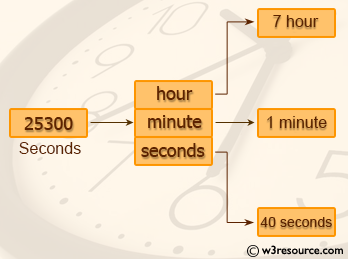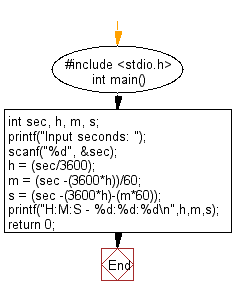﻿ C : Convert a given integer to hours, minutes and seconds

# C Exercises: Convert a given integer to hours, minutes and seconds

## C Basic Declarations and Expressions: Exercise-17 with Solution

Write a C program to convert a given integer (in seconds) to hours, minutes and seconds.

Pictorial Presentation:C Code:

``````#include <stdio.h>
int main() {
int sec, h, m, s; // Declare variables for seconds, hours, minutes, and seconds

// Prompt user for input seconds and store in 'sec'
printf("Input seconds: ");
scanf("%d", &sec);

// Calculate hours, minutes, and remaining seconds
h = (sec/3600);
m = (sec -(3600*h))/60;
s = (sec -(3600*h)-(m*60));

// Print the time in format H:M:S
printf("H:M:S - %d:%d:%d\n",h,m,s);

return 0;
}
``````

Sample Output:

```Input seconds: 25300
H:M:S - 7:1:40
```

Flowchart:C Programming Code Editor:

What is the difficulty level of this exercise?

Test your Programming skills with w3resource's quiz.

﻿